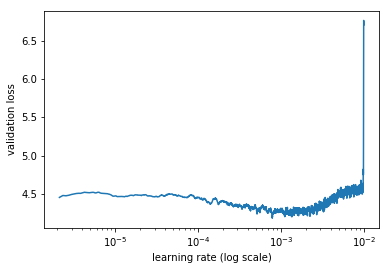# Fat validation loss plot lesson 10

After running `learner.lr_find(start_lr=lrs/10, end_lr=lrs*10, linear=True)` on custom data I got this plot:Does it mean there is no difference between training and validation sets? Something else?

The horizontal y axis says log scale and you used linear=True.

I would guess that linear=True sets the intervals between the different lr in a linear way, i.e. equally spaced, and therefore more points are shown on the right side with a log y axis.

(The other explanation would be that this setup displays the values on a linear y axis with a wrong axis label.)

Maybe you can try it without the linear=True argument to see if this is the cause?

Watched again the lecture. This is the first epoch with custom head, and the lecture’s plot is about the same. Later on with multiple epochs the graph starts to look normal. Will update tomorrow: one epoch takes 2.5 hours and I’m through epoch 2 only.Any updates on this? I am also trying a custom dataset with similar results on the first epoch